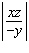Name:    Quiz 1.4-1.5

Multiple Choice
Identify the choice that best completes the statement or answers the question.

Evaluate the expression.

1.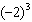a. 8 c. –6 b. –8 d. 6

Multiply.

2.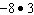a. –24 c. 24 b. –27 d. –21

3.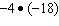a. –72 c. 90 b. 54 d. 72

4.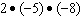a. 79 c. 80 b. –80 d. 83

5.

A manatee dives at a rate of 4 feet per second. What integer represents the change in the manatee’s position after 20 seconds?
 a. 80 c. –60 b. –80 d. –85

6.

You withdraw \$40 each month from your savings account. What integer represents the change in your balance after 4 months?
 a. –120 c. –160 b. 160 d. –200

Evaluate the expression when a = –48, b = 4, and c = –3.

7.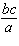a. 0.25 c. –2.75 b. 4 d. –0.25

Divide, if possible.

8.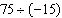a.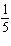c.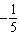b. 5 d. –5

9.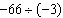a. 22 c. –22 b.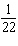d.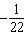Evaluate the expression when a = –50, b = 5, and c = –2.

10.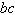a. –10 c. 3 b. 10 d. –52

Numeric Response

Evaluate the expression when x = 12, y = –3, and z = –2.

1.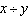2.